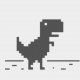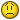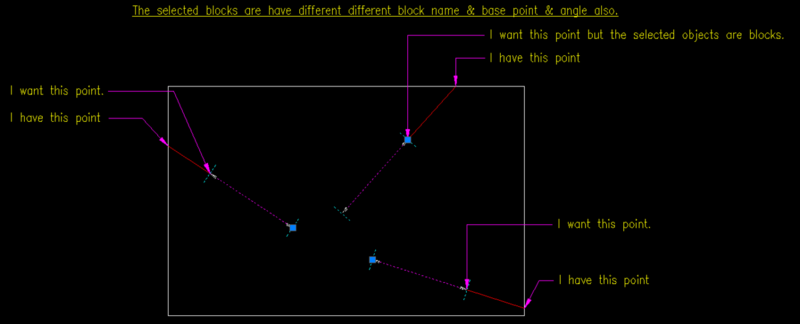# How to get block nearest end point or perpendicular point## Recommended Posts• Replies 35
• Created

#### Top Posters In This Topic

•19

•11

•2

•2

#### Top Posters In This Topic

•Pugazh 19 posts

•dlanorh 11 posts

•Emmanuel Delay 2 posts

•hanhphuc 2 posts

#### Popular PostsHow do we know the length between the block insert point and the point you're looking for?I keep having this same question. From what you're asking I don't know how far that point must be from the block.   Look at this code, made for the dwg attachment. I have differentBlocks don't have endpoints, only insertion points. So lets assume these are dimensions (they are saved as anonymous blocks), is that correct?

#### Posted ImagesHow do we know the length between the block insert point and the point you're looking for?

•1
##### Share on other sites55 minutes ago, Emmanuel Delay said:

How do we know the length between the block insert point and the point you're looking for?

This point taken from i have to make xline from block center point to block insert point then i get from intersection point (xline with outer polyline).

This code is created by @dlanorh, i just modified.

```(defun rh:sammlung_n (o_lst grp / tmp n_lst)
(setq n_lst nil)
(cond ( (and o_lst (= (rem (length o_lst) grp) 0))
(while o_lst
(repeat grp (setq tmp (cons (car o_lst) tmp) o_lst (cdr o_lst)))
(setq n_lst (cons (reverse tmp) n_lst) tmp nil)
);end_while
)
);end_cond
(if n_lst (reverse n_lst))
);end_defun

(defun c:test ( / *error* c_doc c_spc l_obj ent e_lst ss t_obj i_pt t_rot x_obj x_pts s_d x_pt)

(defun *error* ( msg )
(if (not (wcmatch (strcase msg) "*BREAK*,*CANCEL*,*EXIT*")) (princ (strcat "\nOops an Error occurred : " msg)))
(princ)
);end_defun *error*

c_spc (vlax-get-property c_doc (if (= 1 (getvar 'cvport)) 'paperspace 'modelspace))
);end_setq

(while (not l_obj)
(setq ent (car (entsel "\nSelect Line : "))
e_lst (entget ent)
);end_setq
(if (vl-position (cdr (assoc 0 e_lst)) (list "ARC" "LINE" "LWPOLYLINE" "RAY" "SPLINE" "XLINE")) (setq l_obj (vlax-ename->vla-object ent)))
);end_while

(if (and (princ "\nSelect Bars: ")(setq ss (ssget ":L" '((0 . "INSERT")(2 . "`*U*"))))
)

(repeat (setq cnt (sslength ss))
(setq hnd (ssname ss (setq cnd (1- cnt))))
(vla-getboundingbox (vlax-ename->vla-object hnd) 'minpt 'maxpt)
(setq t_obj (vlax-ename->vla-object (ssname ss (setq cnt (1- cnt))))
i_pt (mapcar '/ ; midpoint of bounding box
(mapcar '+ (vlax-safearray->list minpt) (vlax-safearray->list maxpt))
'(2 2 2)
); mapcar
i_pt2 (cdr (assoc 10 (entget hnd))))
(command "_.snapang" "_non" i_pt "_non" i_pt2)
(setq ang (getvar 'snapang))
(setq x_obj (vlax-invoke c_spc 'addxline i_pt (polar i_pt ang 1.0))
x_pts (rh:sammlung_n (vlax-invoke x_obj 'intersectwith l_obj acextendnone) 3)
s_d 1.0e200
);end_setq

(cond ( (> (length x_pts) 1) (foreach pt x_pts (if (< (distance pt i_pt) s_d) (setq x_pt pt s_d (distance pt i_pt)))))
(t (setq x_pt (car x_pts)))
);end_cond

(vla-delete x_obj)
......................
......................
);end_repeat
)
(princ)
);end_defun```

Edited by Pugazh
##### Share on other siteshelp me!!##### Share on other sitesI keep having this same question.

From what you're asking I don't know how far that point must be from the block.

Look at this code, made for the dwg attachment.

I have different blocks, with different rotations, and a rectangle.

My code puts a point on the rectangle.  It also puts a point along that same line at a distance 25 (Assuming your image has a text height of 2.5, those points you want are at that distance)

Command  BFP

```
(defun drawPoint (pt)
(entmakex (list (cons 0 "POINT")
(cons 10 pt)))
)

(defun drawLine (p1 p2)
(entmakex (list (cons 0 "LINE")
(cons 10 p1)
(cons 11 p2)))
)

;;;;;;;;;;;;;;;;;;;;;;;;;;;;;;;;;;;;;;;;;;;;;;;;;;;;;;;;;;;;;;;;;;;;;;;;;;;;;;;;;;;

;; Intersections  -  Lee Mac
;; Returns a list of all points of intersection between two objects
;; for the given intersection mode.
;; ob1,ob2 - [vla] VLA-Objects
;;     mod - [int] acextendoption enum of intersectwith method
;;        acextendnone             Do not extend either object
;;        acextendthisentity       Extend obj1 to meet obj2
;;        acextendotherentity     Extend obj2 to meet obj1
;;        acextendboth             Extend both objects
(defun LM:intersections ( ob1 ob2 mod / lst rtn )
(if (and (vlax-method-applicable-p ob1 'intersectwith)
(vlax-method-applicable-p ob2 'intersectwith)
(setq lst (vlax-invoke ob1 'intersectwith ob2 mod))
)
(repeat (/ (length lst) 3)
lst (cdddr lst)
)
)
)
(reverse rtn)
)

;;;;;;;;;;;;;;;;;;;;;;;;;;;;;;;;;;;;;;;;;;;;;;;;;;;;;;;;;;;;;;;;;;;;;;;;;;;;;;;;;;;

;;
(defun c:bfp ( / rect blocks i l p1 rot p2 p3)
(princ "\nSelect blocks: ")
(setq blocks (ssget (list (cons 0 "INSERT"))))
(setq rect (car (entsel "\nSelect rectangle: ")))
;; I'll extend a line to at least outside the rectangle.  Using the length of the rectangle guarantees this
(setq len (vla-get-length (vlax-ename->vla-object rect)))

(setq i 0)
(repeat (sslength blocks)
;; make a dummy line, we'll erase it later
(setq l (drawLine
(setq p1 (cdr (assoc 10 (entget (ssname blocks i)))))
(polar p1 (setq rot (cdr (assoc 50 (entget (ssname blocks i))))) len)
))
;; find intersect with rectangle
(setq p2 (nth 0 (LM:intersections (vlax-ename->vla-object l) (vlax-ename->vla-object rect) acextendnone)))
(drawPoint p2)
(setq p3 (vlax-curve-getPointAtDist (vlax-ename->vla-object l) 25.0))
(drawPoint p3)
;; delete the line
(entdel l)
(setq i (+ i 1))
)

)

```

Please try to explain again what exactly you need.

•1
##### Share on other sitesThis is i need, how to get block nearest endpoint or perpendicular point from the entsel.

`(list "ARC" "LINE" "LWPOLYLINE" "RAY" "SPLINE" "XLINE")) `

See my attached file, the blocks are selected. did you see block insert point?.  blocks insert point is enough for me but  they blocks are have different rotation.

So i need block endpoint from the entsel.##### Share on other sites7 hours ago, Pugazh said:

This is i need, how to get block nearest endpoint or perpendicular point from the entsel.

```
(list "ARC" "LINE" "LWPOLYLINE" "RAY" "SPLINE" "XLINE")) ```

See my attached file, the blocks are selected. did you see block insert point?.  blocks insert point is enough for me but  they blocks are have different rotation.

So i need block endpoint from the entsel.

Blocks don't have endpoints, only insertion points. So lets assume these are dimensions (they are saved as anonymous blocks), is that correct?

•1
##### Share on other sitesHi

Sorry for the late reply, maybe these are dimensions (they are saved as anonymous blocks). please see the attached sample file.

##### Share on other sitesIannot open this drawing, It needs to be in AutoCAD 2010 format

##### Share on other sites24 minutes ago, dlanorh said:

Iannot open this drawing, It needs to be in AutoCAD 2010 format

##### Share on other sitesTry this. Tested with your block, and will only work with this block. It is an in-elegant short notice solution. The block is composed of a line and four nested blocks. The block is copied, the copy is then exploded and nested blocks removed leaving the line. The end points of the line are tested against the original blocks insertion point and the non-matching coordinate is returned. All created objects are deleted.

```(defun rh:oppend ( obj / n_obj b_objs l_obj s_pt e_pt rtn)
(if (= (type obj) 'ENAME) (setq obj (vlax-ename->vla-object obj)))
(cond ( (= "AcDbBlockReference" (vlax-get obj 'objectname))
(setq n_obj (vla-copy ent)
b_objs (vlax-invoke n_obj 'explode)
l_obj (car (vl-remove-if-not '(lambda (x) (= (vlax-get x 'objectname) "AcDbLine")) b_objs))
s_pt (vlax-get l_obj 'startpoint)
e_pt (vlax-get l_obj 'endpoint)
)
(vla-delete n_obj)
(mapcar '(lambda (x) (vla-delete x)) b_objs)
(if (equal (vlax-get obj 'insertionpoint) s_pt 0.001) (setq rtn e_pt) (setq rtn s_pt))
)
);end_cond
rtn
);end_defun

;; ##### HOW TO USE #####

(setq e_pt (rh:oppend entity)) ; will accept a block entity or a block object. The return (the point you want) will be in the variable e_pt)

```

Edited by dlanorh
##### Share on other sitesOn 21/10/2019 at 11:44, Pugazh said:

This point taken from i have to make xline from block center point to block insert point then i get from intersection point (xline with outer polyline).

This code is created by @dlanorh, i just modified.

```
(defun rh:sammlung_n (o_lst grp / tmp n_lst)
(setq n_lst nil)
(cond ( (and o_lst (= (rem (length o_lst) grp) 0))
(while o_lst
(repeat grp (setq tmp (cons (car o_lst) tmp) o_lst (cdr o_lst)))
(setq n_lst (cons (reverse tmp) n_lst) tmp nil)
);end_while
)
);end_cond
(if n_lst (reverse n_lst))
);end_defun

(defun c:test ( / *error* c_doc c_spc l_obj ent e_lst ss t_obj i_pt t_rot x_obj x_pts s_d x_pt)

(defun *error* ( msg )
(if (not (wcmatch (strcase msg) "*BREAK*,*CANCEL*,*EXIT*")) (princ (strcat "\nOops an Error occurred : " msg)))
(princ)
);end_defun *error*

c_spc (vlax-get-property c_doc (if (= 1 (getvar 'cvport)) 'paperspace 'modelspace))
);end_setq

(while (not l_obj)
(setq ent (car (entsel "\nSelect Line : "))
e_lst (entget ent)
);end_setq
(if (vl-position (cdr (assoc 0 e_lst)) (list "ARC" "LINE" "LWPOLYLINE" "RAY" "SPLINE" "XLINE")) (setq l_obj (vlax-ename->vla-object ent)))
);end_while

(if (and (princ "\nSelect Bars: ")(setq ss (ssget ":L" '((0 . "INSERT")(2 . "`*U*"))))
)

(repeat (setq cnt (sslength ss))
(setq hnd (ssname ss (setq cnd (1- cnt))))
(vla-getboundingbox (vlax-ename->vla-object hnd) 'minpt 'maxpt)
(setq t_obj (vlax-ename->vla-object (ssname ss (setq cnt (1- cnt))))
i_pt (mapcar '/ ; midpoint of bounding box
(mapcar '+ (vlax-safearray->list minpt) (vlax-safearray->list maxpt))
'(2 2 2)
); mapcar
i_pt2 (cdr (assoc 10 (entget hnd))))
(command "_.snapang" "_non" i_pt "_non" i_pt2)
(setq ang (getvar 'snapang))
(setq x_obj (vlax-invoke c_spc 'addxline i_pt (polar i_pt ang 1.0))
x_pts (rh:sammlung_n (vlax-invoke x_obj 'intersectwith l_obj acextendnone) 3)
s_d 1.0e200
);end_setq

(cond ( (> (length x_pts) 1) (foreach pt x_pts (if (< (distance pt i_pt) s_d) (setq x_pt pt s_d (distance pt i_pt)))))
(t (setq x_pt (car x_pts)))
);end_cond

(vla-delete x_obj)
......................
......................
);end_repeat
)
(princ)
);end_defun```

i add this line. it's correct?

`(setq e_pt (rh:oppend hnd))`
Edited by Pugazh
##### Share on other sites7 hours ago, Pugazh said:

i add this line. it's correct?

```
(setq e_pt (rh:oppend hnd))```

Yes, but you don't have to use e_pt as the variable name; you can use whatever variable name makes sense to you. You also need to include the sub function in with the rest of the code.

Edited by dlanorh
##### Share on other sitesi got this Error : bad argument type: VLA-OBJECT

##### Share on other sites1 hour ago, Pugazh said:

i got this Error : bad argument type: VLA-OBJECT

`(setq n_obj (vla-copy ent) `

(vla-copy obj

•1
##### Share on other sites1 hour ago, hanhphuc said:
```
(setq n_obj (vla-copy ent) ```

(vla-copy obj

```(defun rh:oppend ( obj / n_obj b_objs l_obj s_pt e_pt rtn)
(if (= (type obj) 'ENAME) (setq obj (vlax-ename->vla-object obj)))
(cond ( (= "AcDbBlockReference" (vlax-get obj 'objectname))
(setq n_obj (vla-copy obj)
b_objs (vlax-invoke n_obj 'explode)
l_obj (car (vl-remove-if-not '(lambda (x) (= (vlax-get x 'objectname) "AcDbLine")) b_objs))
s_pt (vlax-get l_obj 'startpoint)
e_pt (vlax-get l_obj 'endpoint)
)
(vla-delete n_obj)
(mapcar '(lambda (x) (vla-delete x)) b_objs)
(if (equal (vlax-get obj 'insertionpoint) s_pt 0.001) (setq rtn e_pt) (setq rtn s_pt))
)
);end_cond
rtn
);end_defun```

i just checked

```(setq e_pt (rh:oppend (ssget)))
```

I got same Error : bad argument type: VLA-OBJECT

##### Share on other sites1 hour ago, Pugazh said:

```
(defun rh:oppend ( obj / n_obj b_objs l_obj s_pt e_pt rtn)
(if (= (type obj) 'ENAME) (setq obj (vlax-ename->vla-object obj)))
(cond ( (= "AcDbBlockReference" (vlax-get obj 'objectname))
(setq n_obj (vla-copy obj)
b_objs (vlax-invoke n_obj 'explode)
l_obj (car (vl-remove-if-not '(lambda (x) (= (vlax-get x 'objectname) "AcDbLine")) b_objs))
s_pt (vlax-get l_obj 'startpoint)
e_pt (vlax-get l_obj 'endpoint)
)
(vla-delete n_obj)
(mapcar '(lambda (x) (vla-delete x)) b_objs)
(if (equal (vlax-get obj 'insertionpoint) s_pt 0.001) (setq rtn e_pt) (setq rtn s_pt))
)
);end_cond
rtn
);end_defun```

i just checked

```
(setq e_pt (rh:oppend (ssget)))
```

I got same Error : bad argument type: VLA-OBJECT

It's different time zone, i think @dlanorh will soon reply if he wakes up, so please be patient.

His rh:oppend sub function requires single ENAME or VLA-OBJECT type as argument, not a PICKSET (selectionset)

```
(rh:oppend (car(entsel)) ) ; ENAME

(rh:oppend (ssname (ssget) 0)) ; first item in selection set
```

you can use repeat, while, foreach loop to iterate in selection set.

•1
##### Share on other sites3 hours ago, hanhphuc said:
```
(setq n_obj (vla-copy ent) ```

(vla-copy obj

Thanks, Don't know how I missed that one.

##### Share on other siteshi @dlanorh,

Thank you so muchi think  my question is not possible##### Share on other sites2 hours ago, Pugazh said:

```
(defun rh:oppend ( obj / n_obj b_objs l_obj s_pt e_pt rtn)
(if (= (type obj) 'ENAME) (setq obj (vlax-ename->vla-object obj)))
(cond ( (= "AcDbBlockReference" (vlax-get obj 'objectname))
(setq n_obj (vla-copy obj)
b_objs (vlax-invoke n_obj 'explode)
l_obj (car (vl-remove-if-not '(lambda (x) (= (vlax-get x 'objectname) "AcDbLine")) b_objs))
s_pt (vlax-get l_obj 'startpoint)
e_pt (vlax-get l_obj 'endpoint)
)
(vla-delete n_obj)
(mapcar '(lambda (x) (vla-delete x)) b_objs)
(if (equal (vlax-get obj 'insertionpoint) s_pt 0.001) (setq rtn e_pt) (setq rtn s_pt))
)
);end_cond
rtn
);end_defun```

i just checked

```
(setq e_pt (rh:oppend (ssget)))
```

I got same Error : bad argument type: VLA-OBJECT

You said in an earlier post

Quote

This is i need, how to get block nearest endpoint or perpendicular point from the entsel.

You cannot send it a selection set. As @hanhphuc mentioned you will need to process any selection set in a loop

```(repeat (setq cnt (sslength ss))
(setq e_pt (rh:oppend (ssname ss (setq cnt (1- cnt)))))
;;
;;
;;Rest of your code here to do what you want
;;
;;
);end_repeat

```

I don't know what you are trying to do with the points you have. Perhaps an explanation would help.

## Join the conversation

You can post now and register later. If you have an account, sign in now to post with your account.
Note: Your post will require moderator approval before it will be visible.×   Pasted as rich text.   Restore formatting

Only 75 emoji are allowed.